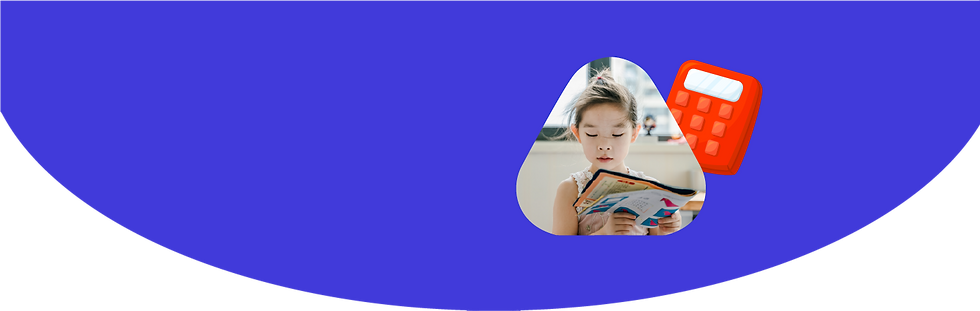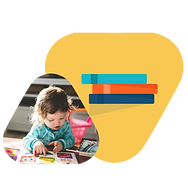Mathematical Literacy is an essential 21st Century Skills

Here’s an exciting chance for you to learn to solve mathematical problems.

Explore World Class CurriculumStrengthen your basics with counting, place values, addition, subtraction, visual analysis and reading time.

1

2

Learn about decimal, fraction, percentage, algebraic expression, geometry, measurement and data handling.1

2

Learn about rational and irrational numbers, ratio ,proportion, coordinate geometry and probability and statistics.###### Curriculum aligned to  USA Common Core ,  UK National  curriculum and 50+ boards across the globe## Personalize the learning pathway of your child.

What To Expect2 Hours Per Week

Our classes meet for 1.5-2hrs per week depending on the age groupThe Classroom

Interactive classes with regular assessments and quizzes.Curriculum

World Class curriculum created by expertsHomework

Continuous assessments and homework to help our students learn better